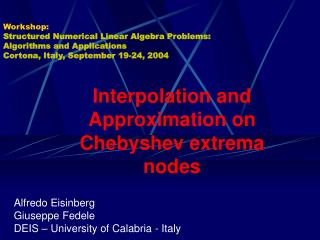DownloadDownload PresentationWorkshop: Structured Numerical Linear Algebra Problems: Algorithms and Applications Cortona, Italy, September 19-24, 200

# Workshop: Structured Numerical Linear Algebra Problems: Algorithms and Applications Cortona, Italy, September 19-24, 200

Download Presentation## Workshop: Structured Numerical Linear Algebra Problems: Algorithms and Applications Cortona, Italy, September 19-24, 200

- - - - - - - - - - - - - - - - - - - - - - - - - - - E N D - - - - - - - - - - - - - - - - - - - - - - - - - - -
##### Presentation Transcript

1. Workshop:Structured Numerical Linear Algebra Problems:Algorithms and ApplicationsCortona, Italy, September 19-24, 2004 Interpolation and Approximation on Chebyshev extrema nodes Alfredo Eisinberg Giuseppe Fedele DEIS – University of Calabria - Italy

2. Outline A property on the elementary symmetric functions Explicit factorization of the inverse of the Vandermonde matrix Symmetric functions for G-L nodes Vandermonde systems on G-L nodes Discrete orthogonal polynomials on G-L nodes Applications

3. A property on elementary symmetric functions

4. A property on elementary symmetric functions Example (continued)

5. A property on elementary symmetric functions A. Eisinberg, C. Picardi On the inversion of Vandermonde matrix IFAC, Kyoto, Japan, 1981. A. Eisinberg, G. Fedele A property on the elementary symmetric functions Unpublished

6. A property on elementary symmetric functions

7. A property on elementary symmetric functions Inverse of the Vandermonde matrix

8. A property on elementary symmetric functions Inverse of the Vandermonde matrix

9. A property on elementary symmetric functions Inverse of the Vandermonde matrix

10. A property on elementary symmetric functions Factorization

11. Chebyshev nodes

12. Chebyshev nodes Standard A. Eisinberg, G. Fedele Polynomial interpolation and related algorithms Twelfth International Colloquium on Num. Anal. And Computer Science with Appl. Plovdiv, 2003.

13. Chebyshev nodes Extended A. Eisinberg, G. Fedele Polynomial interpolation and related algorithms Twelfth International Colloquium on Num. Anal. And Computer Science with Appl. Plovdiv, 2003.

14. Why Gauss-Lobatto nodes? “It has been also shown that the set of nodes coinciding with the Chebyshev extrema, failed to be a good approximation to the optimal interpolation set. Nevertheless, this set of nodes is of considerable interest since as was established by Ehlick and Zeller, the norm of corresponding interpolation operator is less than the norm of the operator (where T is the set of Chebyshev nodes) induced by interpolation at the Chebyshev root. Namely, the following relation holds: ” L. Brutman, A Note on Polynomial Interpolation at the Chebyshev Extrema Nodes, Journal of Approx. Theory 42, 283-292 (1984).

15. Why Gauss-Lobatto nodes? “For some sets of nodes which are of special importance in the interpolation theory, such as equidistant nodes, Chebyshev roots and extrema and others, the behavior of the Lebesgue function is well investigated.” L. Brutman, Lebesgue functions for polynomial interpolation – a survey, Annals of Numerical Mathematics 4, 111-127 (1997).

16. Notes: Chebyshev nodes

17. Chebyshev nodes

18. Interpolation on Gauss-Lobatto nodes

19. Gauss-Lobatto Chebyshev nodes (extrema)

20. Gauss-Lobatto Chebyshev nodes (extrema)

21. Gauss-Lobatto Chebyshev nodes (extrema)

22. Factorization A. Eisinberg, G. Franzè, N. Salerno Rectangular Vandermonde matrices on Chebyshev nodes Linear Algebra Appl., 283 (1998), 205-219.

23. Factorization A. Eisinberg, G. Fedele Vandermonde systems on Gauss-Lobatto Chebyshev nodes Unpublished

24. Algorithm details

25. Algorithm details

26. Algorithm details

27. Algorithm details

28. Numerical experiments Primal system: Dual system:

29. Numerical experiments

30. Numerical experiments

31. Computational cost

32. Frobenius norms

33. Frobenius norms A. Eisinberg, G. Fedele Vandermonde systems on Gauss-Lobatto Chebyshev nodes Unpublished

34. Determinant A. Eisinberg, G. Fedele Vandermonde systems on Gauss-Lobatto Chebyshev nodes Unpublished

35. Discrete orthogonal polynomials on Gauss-Lobatto nodes

36. Discrete orthogonal polynomials on G-L nodes

37. Discrete orthogonal polynomials on G-L nodes A. Eisinberg, G. Fedele Discrete orthogonal polynomials on Gauss-Lobatto Chebyshev nodes Unpublished

38. Discrete orthogonal polynomials on G-L nodes

39. Discrete orthogonal polynomials on G-L nodes

40. Discrete orthogonal polynomials on G-L nodes

41. Discrete orthogonal polynomials on G-L nodes Inner products Three-terms recurrence relation

42. Numerical results

43. Numerical results EF = Our algorithm 4mn flops CB = Conte – De Boor algorithm 10mn flops S. D. Conte, C. De Boor Elementary Numerical Analysis McGraw Hill, 2nd ed., 1972.

44. Numerical results

45. Numerical results

46. Numerical results